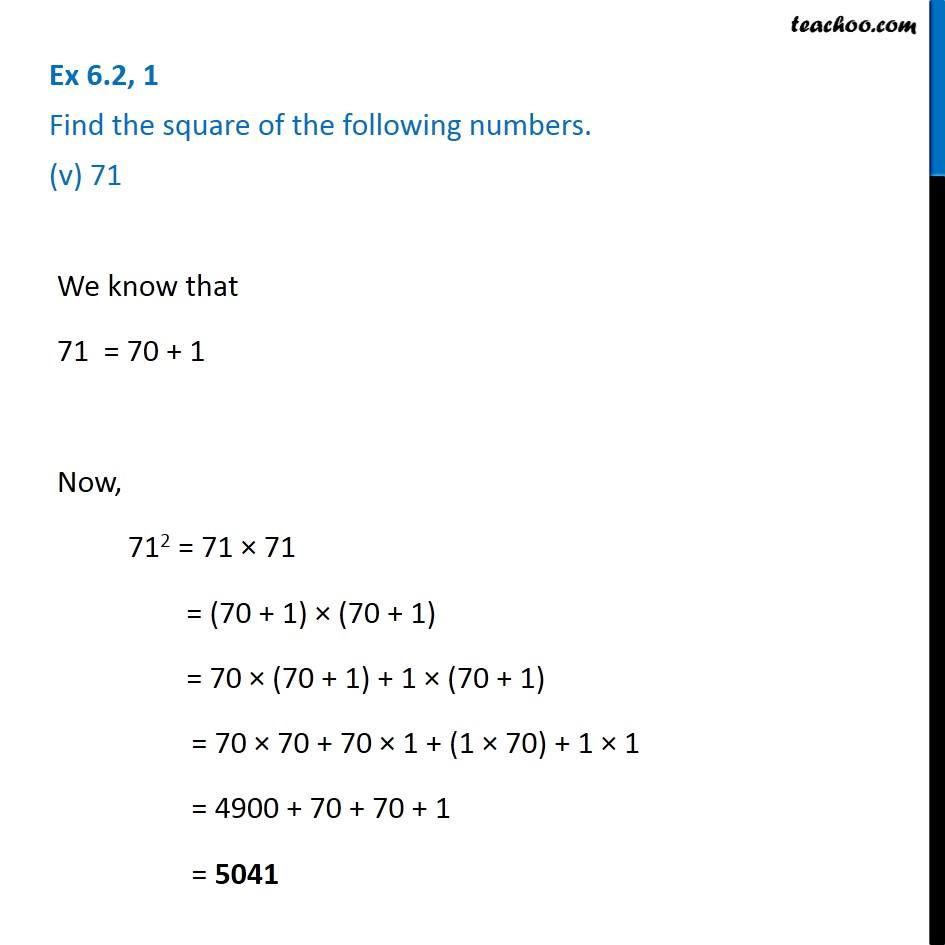Ex 5.2

Chapter 5 Class 8 Squares and Square Roots
Serial order wiseLearn in your speed, with individual attention - Teachoo Maths 1-on-1 Class

### Transcript

Ex 5.2, 1 Find the square of the following numbers. (v) 71We know that 71 = 70 + 1 Now, 712 = 71 × 71 = (70 + 1) × (70 + 1) = 70 × (70 + 1) + 1 × (70 + 1) = 70 × 70 + 70 × 1 + (1 × 70) + 1 × 1 = 4900 + 70 + 70 + 1 = 5041